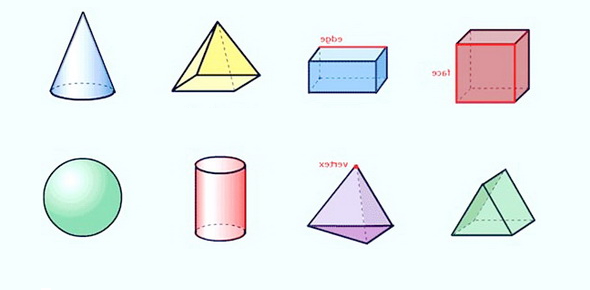# Basic Geometry Practice Test

Approved & Edited by ProProfs Editorial Team
At ProProfs Quizzes, our dedicated in-house team of experts takes pride in their work. With a sharp eye for detail, they meticulously review each quiz. This ensures that every quiz, taken by over 100 million users, meets our standards of accuracy, clarity, and engagement.
| Written by Mathjunkie
M
Mathjunkie
Community Contributor
Quizzes Created: 1 | Total Attempts: 641
Questions: 10 | Attempts: 642SettingsGeometry is a field of mathematics that studies sizes, shapes, positions angles, and dimensions of things. Geometry is one of the oldest and most important branches of mathematics.
This is a mathematical quiz focusing on the basics of geometry. Want to be great at geometry? Want to ace the upcoming geometry exam? Take this quiz and do so!

• 1.

### A rectangle is a square.

• A.

Always

• B.

Sometimes

• C.

Never

B. Sometimes
Explanation
A rectangle is a quadrilateral with four right angles, but it does not necessarily have equal sides. A square, on the other hand, is a special type of rectangle with all sides equal in length. Therefore, while a square can be classified as a rectangle, not all rectangles can be classified as squares. Hence, the statement "A rectangle is a square" is sometimes true, depending on whether the rectangle in question has equal sides or not.

Rate this question:

• 2.

### A square has perpendicular diagonals

• A.

Always

• B.

Sometimes

• C.

Never

A. Always
Explanation
A square is a special type of quadrilateral where all sides are equal in length and all angles are right angles. Perpendicular diagonals are diagonals that intersect at a right angle. In a square, the diagonals are always perpendicular because the opposite sides are parallel and the angles between them are right angles. Therefore, the correct answer is "Always".

Rate this question:

• 3.

### Has perpendicular diagonals

Rhombus, Square, kite
Rh S K
Rhombus square kite
Explanation
All three shapes, the rhombus, square, and kite, have perpendicular diagonals. In a rhombus, the diagonals are perpendicular and bisect each other. In a square, the diagonals are also perpendicular and bisect each other at right angles. In a kite, the diagonals are perpendicular and intersect at one point. Therefore, all three shapes satisfy the criteria of having perpendicular diagonals.

Rate this question:

• 4.

### What are the properties of a rhombus

Has perpendicular diagonals Diagonals bisect the angles Properties 1-5 of Parallelogram Equilateral
Explanation
A rhombus has perpendicular diagonals, meaning that the diagonals intersect at a right angle. The diagonals also bisect the angles of the rhombus, dividing each angle into two equal parts. Additionally, a rhombus has all the properties of a parallelogram, including opposite sides that are parallel and congruent, opposite angles that are congruent, consecutive angles that are supplementary, and diagonals that bisect each other. Finally, a rhombus is also equilateral, meaning that all four sides are congruent in length.

Rate this question:

• 5.

### An isosceles triangle has legs that are congruent

• A.

True

• B.

False

A. True
Explanation
In an isosceles triangle, the two legs are equal in length. This is because an isosceles triangle has two congruent angles, which means that the two sides opposite those angles are also congruent. Therefore, the statement that an isosceles triangle has legs that are congruent is true.

Rate this question:

• 6.

### The circumference of a circle is calculated as

• A.

π times the diameter

• B.

( π times the radius) x 2

• C.

Both

• D.

None of the above

C. Both
Explanation
The correct answer is "Both" because the circumference of a circle can be calculated using either the formula π times the diameter or ( π times the radius) x 2. Both formulas will give you the same result, which is the distance around the circle.

Rate this question:

• 7.

### The Pythagoras theorem is related to which shape?

• A.

Triangle

• B.

Rectangle

• C.

Circle

• D.

All of the above

A. Triangle
Explanation
The Pythagoras theorem states that in a right-angled triangle, the square of the length of the hypotenuse is equal to the sum of the squares of the lengths of the other two sides. This theorem is specifically related to triangles, as it helps in finding the length of one side of a right-angled triangle when the lengths of the other two sides are known. Therefore, the correct answer is Triangle.

Rate this question:

• 8.

### Perpendicular is the shortest distance between a line and a point

• A.

True

• B.

False

• C.

Sometimes

A. True
Explanation
The statement is true because in geometry, the perpendicular line is defined as the line that forms a 90-degree angle with another line or surface. When a line is perpendicular to a point, it forms the shortest distance between the line and the point. This can be visualized by imagining a line and a point, and drawing a line perpendicular to the original line that passes through the given point. The perpendicular line will be the shortest distance between the original line and the point.

Rate this question:

• 9.

### A _____ has 0 vertices and 0 edges.

Circle
Explanation
A circle is a closed curve that is formed by all points in a plane that are equidistant from a fixed center point. It has no vertices because it is a smooth, continuous curve without any sharp corners or points. Additionally, a circle has no edges because it is not made up of line segments. Instead, it is a single, unbroken curve. Therefore, a circle has 0 vertices and 0 edges.

Rate this question:

• 10.

### A ____ has two pairs of equal adjacent sides.Back to top